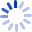Regression Analysis by Example

The essentials of regression analysis through practical applications Regression analysis is a conceptually simple method for investigating relationships among variables. Carrying out a successful application of regression analysis, however, requires a balance of theoretical results, empirical rules, and subjective judgement. Regression Analysis by Example, Fourth Edition has been expanded and thoroughly updated to reflect recent advances in the field. The emphasis continues to be on exploratory data analysis rather than ...

Cash for Textbooks